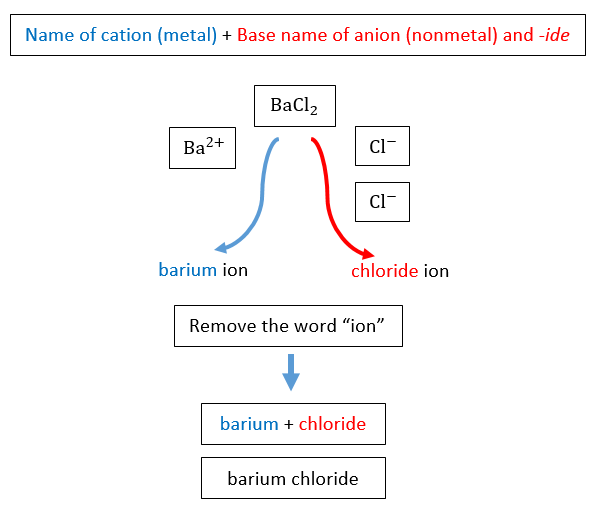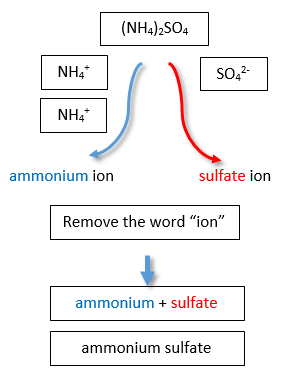# 5.7: Naming Ionic Compounds

$$\newcommand{\vecs}{\overset { \rightharpoonup} {\mathbf{#1}} }$$ $$\newcommand{\vecd}{\overset{-\!-\!\rightharpoonup}{\vphantom{a}\smash {#1}}}$$$$\newcommand{\id}{\mathrm{id}}$$ $$\newcommand{\Span}{\mathrm{span}}$$ $$\newcommand{\kernel}{\mathrm{null}\,}$$ $$\newcommand{\range}{\mathrm{range}\,}$$ $$\newcommand{\RealPart}{\mathrm{Re}}$$ $$\newcommand{\ImaginaryPart}{\mathrm{Im}}$$ $$\newcommand{\Argument}{\mathrm{Arg}}$$ $$\newcommand{\norm}{\| #1 \|}$$ $$\newcommand{\inner}{\langle #1, #2 \rangle}$$ $$\newcommand{\Span}{\mathrm{span}}$$ $$\newcommand{\id}{\mathrm{id}}$$ $$\newcommand{\Span}{\mathrm{span}}$$ $$\newcommand{\kernel}{\mathrm{null}\,}$$ $$\newcommand{\range}{\mathrm{range}\,}$$ $$\newcommand{\RealPart}{\mathrm{Re}}$$ $$\newcommand{\ImaginaryPart}{\mathrm{Im}}$$ $$\newcommand{\Argument}{\mathrm{Arg}}$$ $$\newcommand{\norm}{\| #1 \|}$$ $$\newcommand{\inner}{\langle #1, #2 \rangle}$$ $$\newcommand{\Span}{\mathrm{span}}$$$$\newcommand{\AA}{\unicode[.8,0]{x212B}}$$

##### Learning Objectives
• To use the rules for naming ionic compounds.

After learning a few more details about the names of individual ions, you will be one step away from knowing how to name ionic compounds. This section begins the formal study of nomenclature, the systematic naming of chemical compounds.

## Naming Ions

The name of a monatomic cation is simply the name of the element followed by the word ion. Thus, Na+ is the sodium ion, Al3+ is the aluminum ion, Ca2+ is the calcium ion, and so forth.

We have seen that some elements lose different numbers of electrons, producing ions of different charges (Figure 3.3). Iron, for example, can form two cations, each of which, when combined with the same anion, makes a different compound with unique physical and chemical properties. Thus, we need a different name for each iron ion to distinguish Fe2+ from Fe3+. The same issue arises for other ions with more than one possible charge.

There are two ways to make this distinction. In the simpler, more modern approach, called the Stock system, an ion’s positive charge is indicated by a roman numeral in parentheses after the element name, followed by the word ion. Thus, Fe2+ is called the iron(II) ion, while Fe3+ is called the iron(III) ion. This system is used only for elements that form more than one common positive ion. We do not call the Na+ ion the sodium(I) ion because (I) is unnecessary. Sodium forms only a 1+ ion, so there is no ambiguity about the name sodium ion.

Table $$\PageIndex{1}$$: The Modern and Common System of Cation Names
Element Stem Charge Modern Name Common Name
iron ferr- 2+ iron(II) ion ferrous ion
3+ iron(III) ion ferric ion
copper cupr- 1+ copper(I) ion cuprous ion
2+ copper(II) ion cupric ion
tin stann- 2+ tin(II) ion stannous ion
4+ tin(IV) ion stannic ion
chromium chrom- 2+ chromium(II) ion chromous ion
3+ chromium(III) ion chromic ion
gold aur- 1+ gold(I) ion aurous ion
3+ gold(III) ion auric ion

The second system, called the common system, is not conventional but is still prevalent and used in the health sciences. This system recognizes that many metals have two common cations. The common system uses two suffixes (-ic and -ous) that are appended to the stem of the element name. The -ic suffix represents the greater of the two cation charges, and the -ous suffix represents the lower one. In many cases, the stem of the element name comes from the Latin name of the element. Table $$\PageIndex{1}$$ lists the elements that use the common system, along with their respective cation names.

Table $$\PageIndex{2}$$: Some Monatomic Anions
Ion Name
F fluoride ion
Cl chloride ion
Br bromide ion
I iodide ion
O2− oxide ion
S2− sulfide ion
P3− phosphide ion
N3− nitride ion

The name of a monatomic anion consists of the stem of the element name, the suffix -ide, and then the word ion. Thus, as we have already seen, Cl is “chlor-” + “-ide ion,” or the chloride ion. Similarly, O2− is the oxide ion, Se2 is the selenide ion, and so forth. Table $$\PageIndex{2}$$ lists the names of some common monatomic ions. The polyatomic ions have their own characteristic names, as discussed earlier.

##### Example $$\PageIndex{1}$$

Name each ion.

1. Ca2+
2. S2−
3. SO32
4. NH4+
5. Cu+
###### Solution
1. the calcium ion
2. the sulfide ion
3. the sulfite ion
4. the ammonium ion
5. the copper(I) ion or the cuprous ion
##### Exercise $$\PageIndex{1}$$

Name each ion.

1. Fe2+
2. Fe3+
3. SO42
4. Ba2+
5. HCO3
iron(II) ion
iron(III) ion
sulfate ion
barium ion
hydrogen carbonate ion or bicarbonate ion
##### Example $$\PageIndex{2}$$

Write the formula for each ion.

1. the bromide ion
2. the phosphate ion
3. the cupric ion
4. the magnesium ion
1. Br
2. PO43
3. Cu2+
4. Mg2+
##### Exercise $$\PageIndex{2}$$

Write the formula for each ion.

1. the fluoride ion
2. the carbonate ion
3. the stannous ion
4. the potassium ion
F-
CO32-
Sn 2+
K+

## Naming Binary Ionic Compounds with a Metal that Forms Only One Type of Cation

A binary ionic compound is a compound composed of a monatomic metal cation and a monatomic nonmetal anion. The metal cation is named first, followed by the nonmetal anion as illustrated in Figure $$\PageIndex{1}$$ for the compound BaCl2. The word ion is dropped from both parts.Figure $$\PageIndex{1}$$: Naming $$BaCl_2$$ Naming formula: Name of metal cation + base name of nonmetal anion + suffix -ide. BaCl2 is named as barium chloride.

Subscripts in the formula do not affect the name.

##### Example $$\PageIndex{3}$$: Naming Ionic Compounds

Name each ionic compound.

1. CaCl2
2. AlF3
3. KCl
###### Solution
1. Using the names of the ions, this ionic compound is named calcium chloride.
2. The name of this ionic compound is aluminum fluoride.
3. The name of this ionic compound is potassium chloride
##### Exercise $$\PageIndex{3}$$

Name each ionic compound.

1. AgI
2. MgO
3. Ca3P2
silver iodide
magnesium oxide
calcium phosphide

## Naming Binary Ionic Compounds with a Metal That Forms More Than One Type of Cation

If you are given a formula for an ionic compound whose cation can have more than one possible charge, you must first determine the charge on the cation before identifying its correct name. For example, consider FeCl2 and FeCl3 . In the first compound, the iron ion has a 2+ charge because there are two Cl ions in the formula (1− charge on each chloride ion). In the second compound, the iron ion has a 3+ charge, as indicated by the three Cl ions in the formula. These are two different compounds that need two different names. By the Stock system, the names are iron(II) chloride and iron(III) chloride (Figure $$\PageIndex{2}$$).

Table $$\PageIndex{3}$$: Naming the $$FeCl_2$$ and $$FeCl_3$$ Compounds in the Modern/Stock System.
Name of cation (metal) + (Roman Numeral in parenthesis) + Base name of anion (nonmetal) and -ideIf we were to use the stems and suffixes of the common system, the names would be ferrous chloride and ferric chloride, respectively (Figure $$\PageIndex{3}$$) .

Table $$\PageIndex{4}$$: Naming the $$FeCl_2$$ and $$FeCl_3$$ Compounds in the Old/Common System.
"Old" base name of cation (metal) and -ic or -ous + Base name of anion (nonmetal) and -ide

-ous (for ions with lower charge)-ic (for ions with higher charge)##### Example $$\PageIndex{4}$$:

Name each ionic compound.

1. Co2O3
2. FeCl2
###### Solution
Solutions to Example 5.7.4
a

We know that cobalt can have more than one possible charge; we just need to determine what it is.

• Oxide always has a 2− charge, so with three oxide ions, we have a total negative charge of 6−.
• This means that the two cobalt ions have to contribute 6+, which for two cobalt ions means that each one is 3+.
• Therefore, the proper name for this ionic compound is cobalt(III) oxide.
cobalt(III) oxide
b

Iron can also have more than one possible charge.

• Chloride always has a 1− charge, so with two chloride ions, we have a total negative charge of 2−.
• This means that the one iron ion must have a 2+ charge.
• Therefore, the proper name for this ionic compound is iron(II) chloride.
iron(II) chloride
##### Exercise $$\PageIndex{4}$$

Name each ionic compound.

1. AuCl3
2. PbO2
3. CuO
gold(III) chloride
copper(II) oxide

## Naming Ionic Compounds with Polyatomic Ions

The process of naming ionic compounds with polyatomic ions is the same as naming binary ionic compounds. The cation is named first, followed by the anion. One example is the ammonium sulfate compound in Figure $$\PageIndex{6}$$.Figure $$\PageIndex{2}$$: Naming Ionic Compounds with Polyatomic Ions
##### Example $$\PageIndex{5}$$: Naming Ionic Compounds

Write the proper name for each ionic compound.

1. (NH4)2S
2. AlPO4,
3. Fe3(PO4)2
###### Solution
Solutions to Example 5.7.5

a. The ammonium ion has a 1+ charge and the sulfide ion has a 2− charge.

Two ammonium ions need to balance the charge on a single sulfide ion.

The compound’s name is ammonium sulfide.

ammonium sulfide

b. The ions have the same magnitude of charge, one of each (ion) is needed to balance the charges.

The name of the compound is aluminum phosphate.

aluminum phosphate

c. Neither charge is an exact multiple of the other, so we have to go to the least common multiple of 6.

To get 6+, three iron(II) ions are needed, and to get 6−, two phosphate ions are needed .

The compound’s name is iron(II) phosphate.

iron(II) phosphate
##### Exercise $$\PageIndex{5A}$$

Write the proper name for each ionic compound.

1. (NH4)3PO4
2. Co(NO2)3
ammonium phosphate
cobalt(III) nitrite

Figure $$\PageIndex{1}$$ is a synopsis of how to name simple ionic compounds.Figure $$\PageIndex{3}$$: A Guide to Naming Simple Ionic Compounds.
##### Exercise $$\PageIndex{5B}$$

Name each ionic compound.

1. ZnBr2
2. Al2O3
3. (NH4)3PO4
4. AuF3
5. AgF
zinc bromide
aluminum oxide
ammonium phosphate
gold(III) fluoride or auric fluoride